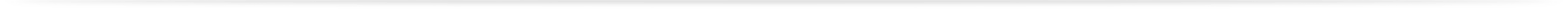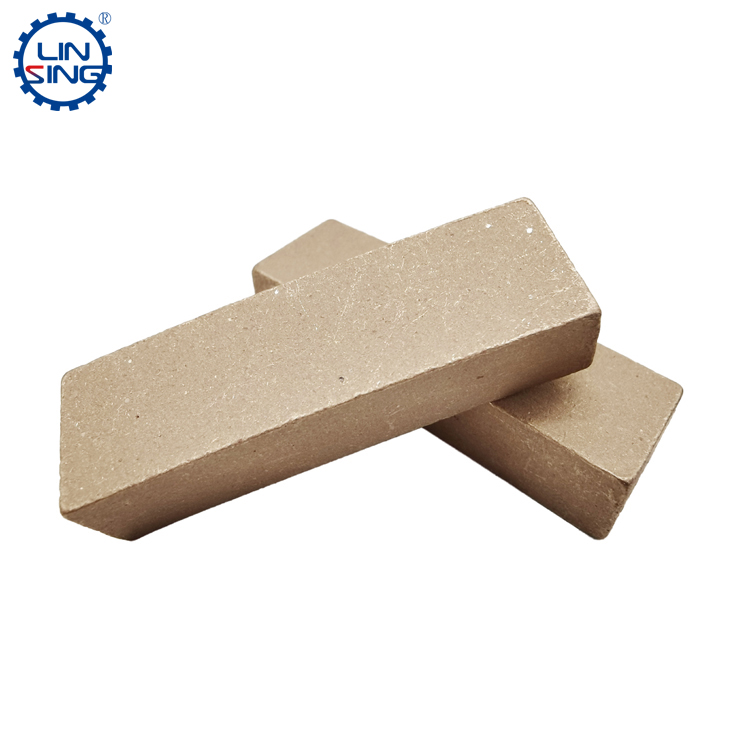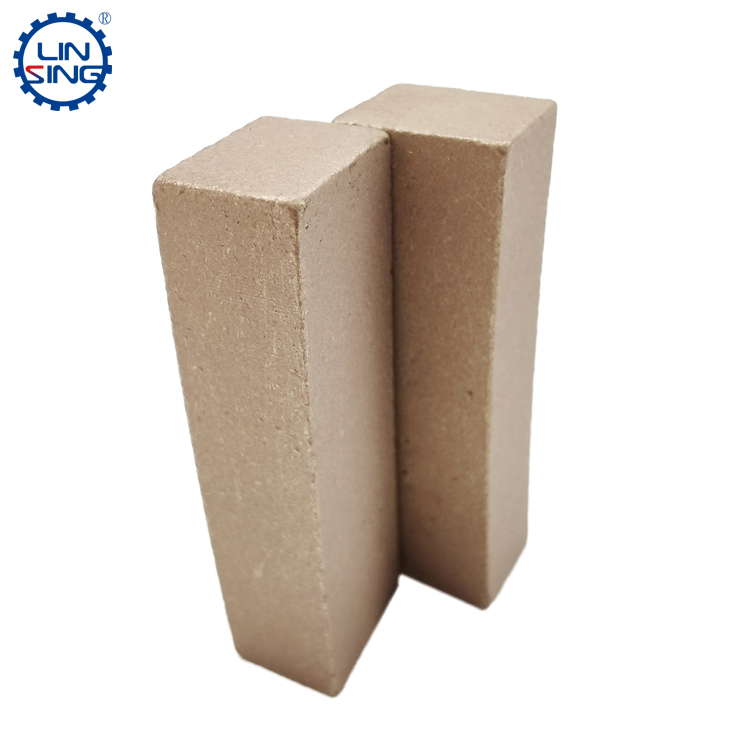# Diamond segment volume calculation

Publish date：2022-07-13 16:47:09 Article From：Linsing Diamond Tools Clicks：

When it comes to the volume of the segment, many factories rarely measure the volume of the segment, because each segment has weight and volume, with these two parameters, we can calculate the density of the segment in many ways. Density is a very important quality parameter for the segment. The more stable the segment, the higher the density. Conversely, the lower the segment density, the structure of the segment is generally not stable.

There are many types of segments, and there are many different situations in the way of measuring the volume due to the different shapes. First of all, take the flat segment. The volume of this segment is very special, because the upper and lower sides are the same width. Therefore, the method of measuring the volume is relatively simple. We learned in elementary school: length multiplied by width multiplied by height, which is the actual volume of the segment.In the second type, we measure the upper width and lower width of the flat segment for marble. The height of this segment is the same. The only difference is the area of the upper and lower sides. At this time, we only need to add the upper area to the lower area. Divide by two and multiply by the height of this segment, then the calculation of the segment volume can be completed.

The third is that we measure the M-shaped granite segment. There are two heights of this segment (because the M-shaped segment has the highest point and the lowest point), and there are also two widths, so we need to ask for the height difference between the highest point and the lowest point, then use the lowest height plus half of the height difference, which is the height of the entire segment. In terms of width, divide the bottom width plus the bottom width by two to get the average width. Then finally multiply the average width by the length and multiply the height of the segment, and finally find the volume of the segment.

The fourth is the saw blade segment. The circle of the saw blade is taken as the center point, and the area difference between the inside and outside of the blade is calculated. Finally, multiply by the thickness of the blade to calculate the volume of the saw blade.The fifth type is a gang saw segment. The gang saw segment is a special kind of segment with a wide upper part and a narrow lower part. The whole shape looks like a trapezoid, no matter from the front view or the side view, it is a trapezoid. The calculation method of this double trapezoid three-dimensional structure is not complicated, that is to get the middle value of the trapezoid length and width, and finally multiply by the height of the segment, you can find the volume of such a gang saw segment.

The most difficult to calculate is the turbine-type segment, and some turbine segments also use the shape of a single-sided turbine when designing, which is very trouble to calculate. For this type of segment, it is best to put it into the water to find the volume of the overflowing water. It is faster, and this method is also widely used in other segments. However, due to the tension of water, it is difficult to accurately measure the exact volume of a segment, and multiple segments can be put in. The more the number, the more accurate the measured result will be. There are many ways to measure the volume of the segment, such as the measurement of the mold groove, which are not listed here.

### Related News